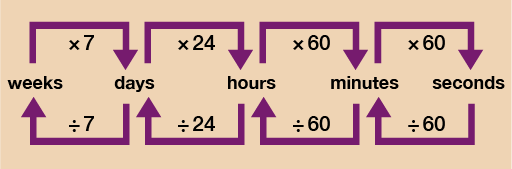Science, Maths & Technology

### Become an OU studentEveryday maths 2

Start this free course now. Just create an account and sign in. Enrol and complete the course for a free statement of participation or digital badge if available.

# 3.2 Converting units of time

You can see from the diagram below that to convert units of time you can use a very similar method to the one you used when converting other units of measure. There is one slight difference when working with time however.Figure 17 A conversion chart for time

Let’s say you want to work out how long 245 minutes is in hours. The diagram above shows that you should do 245 ÷ 60 = 4.083. This is not a particularly helpful answer since you really want the answer in the format of: ___ hours __ minutes. Due to the fact that time does not work in 10s, you need to do a little more work once arriving at your answer of 4.083.

The answer is obviously 4 hours and an amount of minutes.

4 hours then is 4 × 60 = 240 minutes.

Since you wanted to know how long 245 minutes is you just do 245 – 240 = 5 minutes left over. So 245 minutes is 4 hours and 5 minutes.

It’s a very similar process if you want to go from say minutes to seconds. Let’s take it you want to know how long 5 minutes and 17 seconds is in seconds. 5 minutes would be 5 × 60 = 300 seconds. You then have a further 17 seconds to add on so you do 300 + 17 = 317 seconds.

Have a go at the activity below to make sure you feel confident with converting times.

## Activity 6: Converting times

Convert the following times:

• a.6 hours and 35 minutes = ___ minutes.
• b.85 minutes = ____ hours and ____ minutes.
• c.153 seconds = ____ minutes and ___ seconds.
• d.46 days = ___ weeks and ____days.
• e.3 minutes and 40 seconds = ____ seconds.

• a.6 hours = 6 × 60 = 360 minutes

360 minutes + 35 minutes = 395 minutes

• b.85 minutes ÷ 60 = 1.416

1 hour = 60 minutes.

85 minutes − 60 minutes = 25 minutes remaining

So 85 minutes = 1 hour and 25 minutes

• c.153 seconds ÷ 60 = 2.55

2 minutes = 2 × 60 = 120 seconds

153 seconds − 120 seconds = 33 seconds remaining

So 153 seconds = 2 minutes and 33 seconds

• d.46 days ÷ 7 = 6.571…

6 weeks = 6 × 7 = 42 days

46 days − 42 days = 4 days remaining

So 46 days = 6 weeks and 4 days

• e.3 minutes = 3 × 60 = 180 seconds

180 seconds + 40 seconds = 220 seconds

Hopefully you found that activity fairly straightforward and are now feeling ready to move on to the last part of the ‘Units of measure’ session – Average speed.